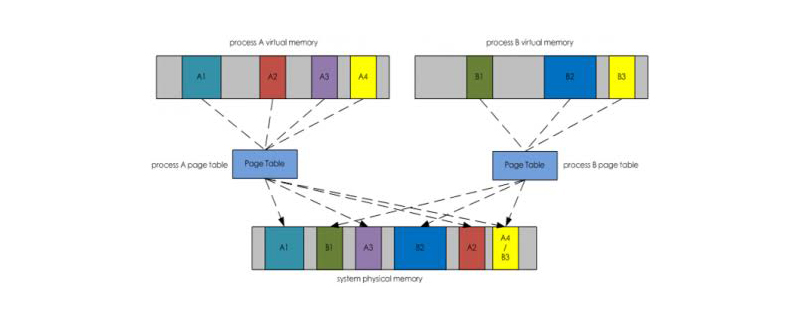# 一图说清Linux中的虚拟内存、驻留内存、共享内存

## 几种内存概念### 虚拟内存

• A进程的 A1+A2+A3+A4+灰色部分=A进程虚拟内存大小

• B进程的 B1+B2+B3+灰色部分=B进程虚拟内存大小

### 共享内存

• A 进程的 A4 = A 进程共享内存

• B 进程的 B3 = B 进程共享内存

### 驻留内存

• A 进程的 A1+A2+A3+A4 = A进程的驻留内存

• B 进程的 B1+B2+B3 = B进程的驻留内存